# Bell number diagrams

The Bell numbers (1, 1, 2, 5, 15, 52, 203, 877, 4140, 21147, 115975, 678570, 4213597, ...) describe the number of ways a set with n elements can be partitioned into disjoint, non-empty subsets.

For example, the set {1, 2, 3} can be partitioned in the following ways:

• {{1}, {2}, {3}}
• {{1, 2}, {3}}
• {{1, 3}, {2}}
• {{1}, {2, 3}}
• {{1, 2, 3}}.

The nth Bell number can be computed using the formula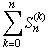,
where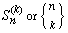represents the Stirling numbers of the second kind.

Here are some diagrams representing the different ways the sets can be partitioned: a line connects elements in the same subset, and a point represents a singleton subset.

(See below for Mathematica code to generate the lists of partitions.)

{1, 2, 3}, 5 partitions:{1, 2, 3, 4}, 15 partitions: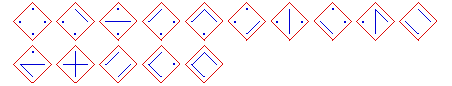{1, 2, 3, 4, 5}, 52 partitions: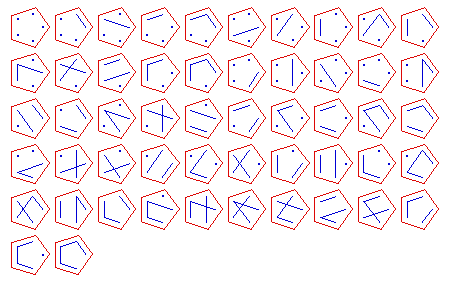{1, 2, 3, 4, 5, 6}, 203 partitions: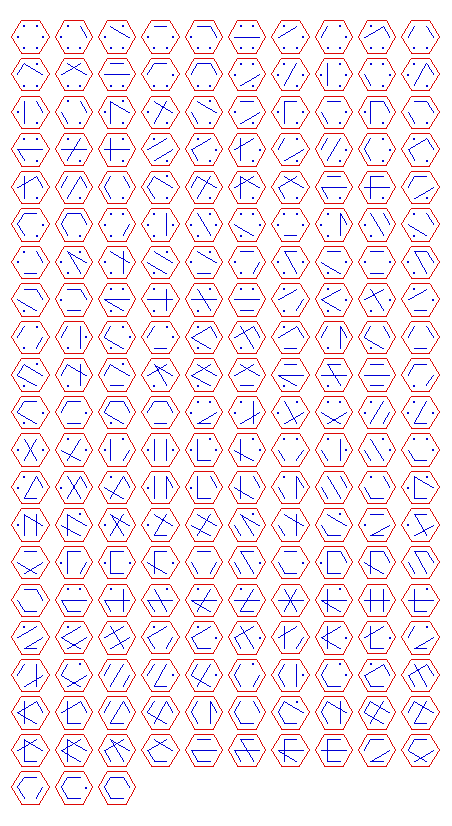{1, 2, 3, 4, 5, 6, 7}, 877 partitions:
(The GIF image is about 125K, so let me know if you're dying to see it.)

Here's some Mathematica 3.0 code to generate the lists of Bell partitions. In short, the program computes lists of partitions recursively, using two cases. We compute BellList[n] by appending the singleton {n} to each element of BellList[n-1], and by appending the number n to each subset of each element in BellList[n-1]; this technique corresponds to the summation of the recurrence for Stirling numbers of the second kind: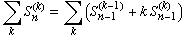.
```BellList = {{{1}}}; (* the basic case *)

BellList[n_Integer?Positive] := BellList[n] = (* do some caching *)

Flatten[
Join[
Map[ReplaceList[#,{S__}->{S,{n}}]&, BellList[n-1]],

Map[ReplaceList[#,{b___,{S__},a___}->{b,{S,n},a}]&, BellList[n-1]]],
1]
```
Some samples:
```BellList//ColumnForm

{{1}, {2}}
{{1, 2}}

BellList//ColumnForm

{{1}, {2}, {3}}
{{1, 2}, {3}}
{{1, 3}, {2}}
{{1}, {2, 3}}
{{1, 2, 3}}

BellList//ColumnForm

{{1}, {2}, {3}, {4}}
{{1, 2}, {3}, {4}}
{{1, 3}, {2}, {4}}
{{1}, {2, 3}, {4}}
{{1, 2, 3}, {4}}
{{1, 4}, {2}, {3}}
{{1}, {2, 4}, {3}}
{{1}, {2}, {3, 4}}
{{1, 2, 4}, {3}}
{{1, 2}, {3, 4}}
{{1, 3, 4}, {2}}
{{1, 3}, {2, 4}}
{{1, 4}, {2, 3}}
{{1}, {2, 3, 4}}
{{1, 2, 3, 4}}
```
Designed and rendered using Mathematica 3.0 for the Apple Macintosh.